# The Tetrakis Hexahedron

The tetrakis hexahedron is a 3D Catalan solid bounded by 24 isosceles triangles, 36 edges (24 short, 12 long), and 14 vertices. It is the dual of the truncated octahedron.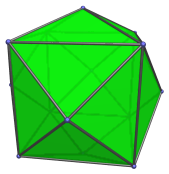The triangular faces are transitive, with two short edges and a long edge with a 3 : 4 length ratio. The 14 vertices are of two kinds: 6 apical vertices corresponding to an inscribed octahedron, and 8 vertices corresponding to an inscribed cube.

The tetrakis hexahedron is the projection envelope of the 24-cell in its vertex-first perspective projection into 3D.

## Projections

The following are images of the tetrakis hexahedron from various viewpoints:

Projection Description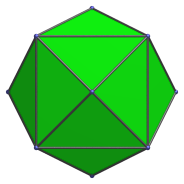Front view, centered on an apical vertex.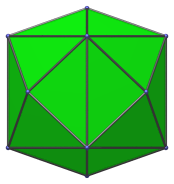Side view, centered on a long edge.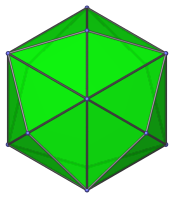Centered on a cube vertex.

## Animation

Here's an animation of a tetrakis hexahedron rotating around the vertical axis: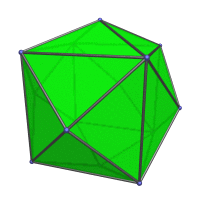## Coordinates

The Cartesian coordinates for the tetrakis hexahedron are all permutations of coordinate and changes of sign of:

• (0, 0, 3)
• (2, 2, 2)

These coordinates can be obtained by inverting a truncated octahedron of edge length √2/6.

Last updated 01 Mar 2023.